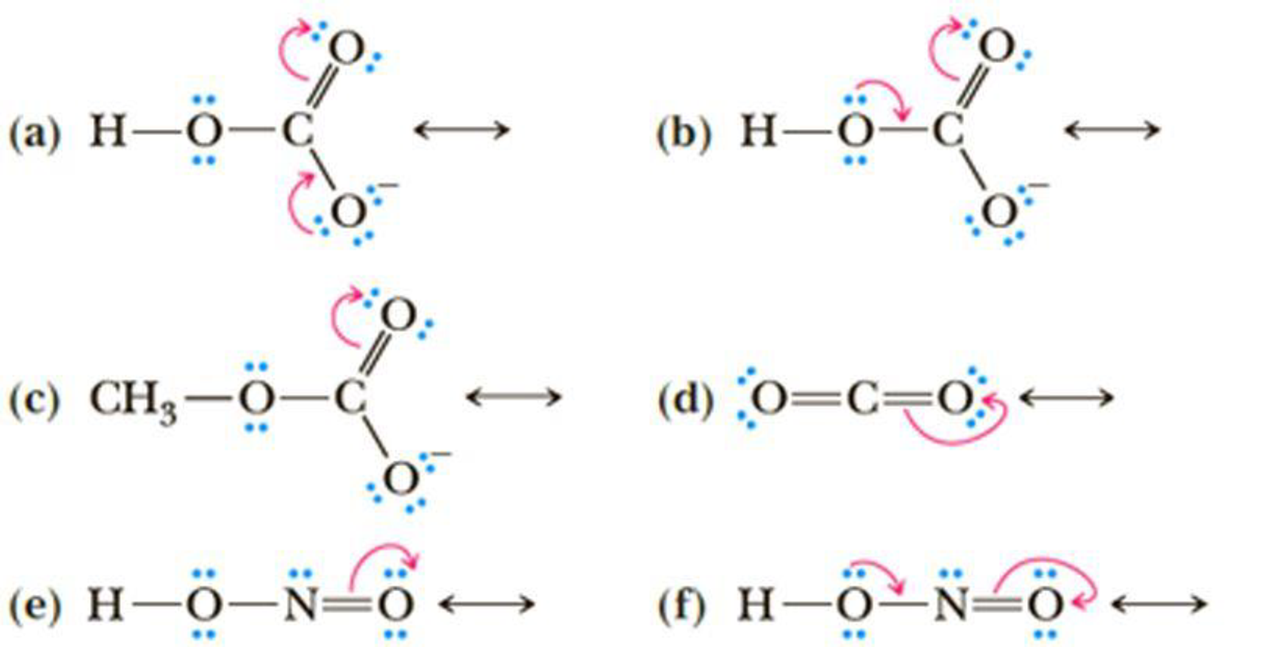# Draw the contributing structure indicated by the curved arrow(s). Assign formal charges as appropriate.### Organic Chemistry

8th Edition
William H. Brown + 3 others
Publisher: Cengage Learning
ISBN: 9781305580350

#### Solutions

Chapter
Section### Organic Chemistry

8th Edition
William H. Brown + 3 others
Publisher: Cengage Learning
ISBN: 9781305580350
Chapter 1, Problem 1.52P
Textbook Problem
1 views

## Draw the contributing structure indicated by the curved arrow(s). Assign formal charges as appropriate.(a)

Interpretation Introduction

Interpretation:

The curve arrow should be drawn for contributing structure and all formal charges have to be shown.

Concept introduction:

Resonance: The delocalization of electrons which is characterized as several structural changes.

### Explanation of Solution

The curve arrow is drawn for contributing structure and all formal charges is shown below.

(b)

Interpretation Introduction

Interpretation:

The curve arrow should be drawn for contributing structure and all formal charges has to be shown.

Concept introduction:

Resonance: The delocalization of electrons which is characterized as several structural changes.

(c)

Interpretation Introduction

Interpretation:

The curve arrow should be drawn for contributing structure and all formal charges has to be shown.

Concept introduction:

Resonance: The delocalization of electrons which is characterized as several structural changes.

(d)

Interpretation Introduction

Interpretation:

The curve arrow should be drawn for contributing structure and all formal charges has to be shown.

Concept introduction:

Resonance: The delocalization of electrons which is characterized as several structural changes.

(e)

Interpretation Introduction

Interpretation:

The curve arrow should be drawn for contributing structure and all formal charges has to be shown.

Concept introduction:

Resonance: The delocalization of electrons which is characterized as several structural changes.

(e)

Interpretation Introduction

Interpretation:

The curve arrow should be drawn for contributing structure and all formal charges has to be shown.

Concept introduction:

Resonance: The delocalization of electrons which is characterized as several structural changes.

### Still sussing out bartleby?

Check out a sample textbook solution.

See a sample solution

#### The Solution to Your Study Problems

Bartleby provides explanations to thousands of textbook problems written by our experts, many with advanced degrees!

Get Started

Find more solutions based on key concepts
Imagine you have 1 kg each of iron, glass, and water, and all three samples are at 10C. (a) Rank the samples fr...

Physics for Scientists and Engineers, Technology Update (No access codes included)

Why did the Copernican hypothesis win gradual acceptance?

Horizons: Exploring the Universe (MindTap Course List)

Under certain circumstances, amino acids can be converted to glucose and so serve the energy needs of the brain...

Nutrition: Concepts and Controversies - Standalone book (MindTap Course List)

The metric prefix micro- means ___. (1.5)

An Introduction to Physical Science